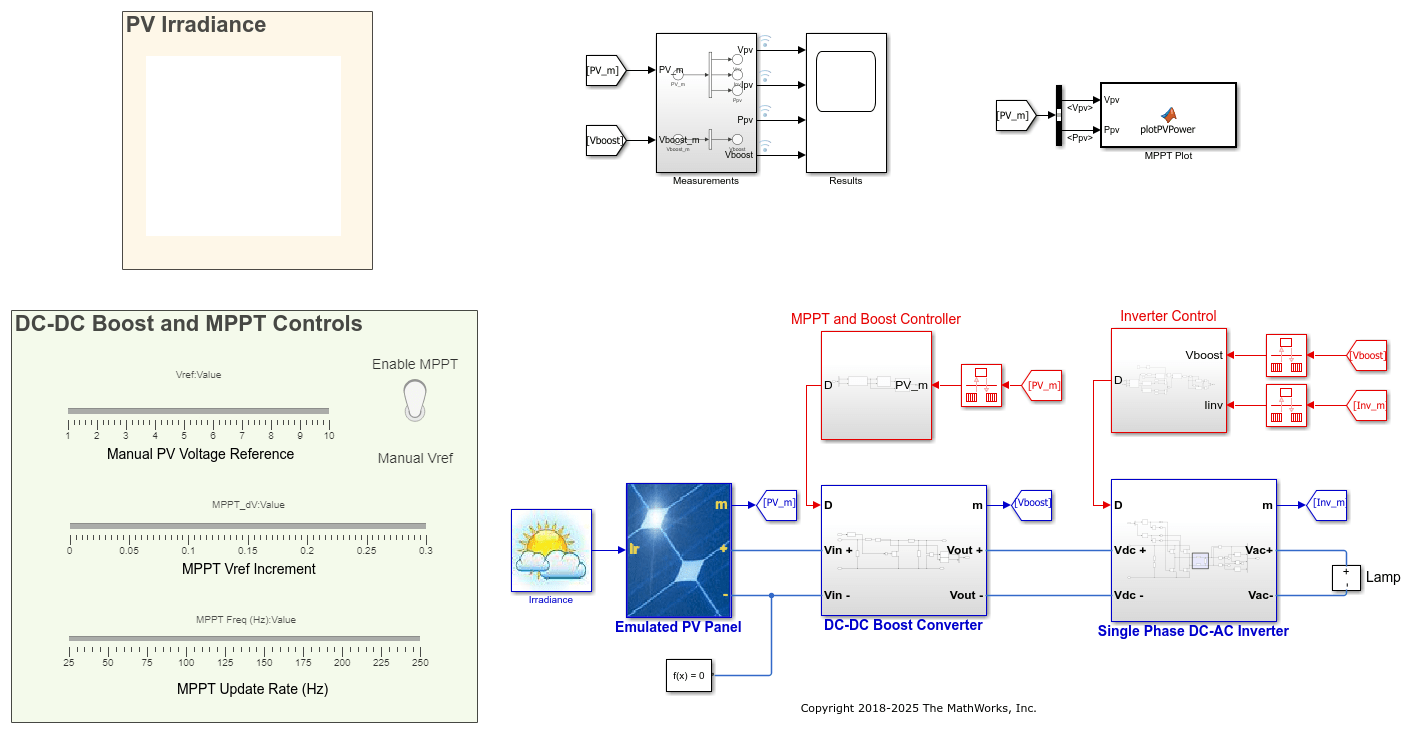# Photovoltaic Inverter with MPPT Using Solar Explorer Kit

This example shows how to implement a photovoltaic (PV) inverter system using the C2000™ Microcontroller Blockset. The example uses the Texas Instruments Solar Explorer Kit along with the Texas Instruments F28035 controlCARD.

Using this example, you can:

• Simulate a plant model for a PV inverter system

• Test the performance and tune the control algorithm

• Generate code for the controller and load it on the controlCARD

• Monitor signals and tune parameters using the host computer

### Required Hardware

• Texas Instruments Solar Explorer Kit (TMDSSOLAR(P/C)EXPKIT)

• F28035 controlCARD

### Simulate the Photovoltaic Inverter with MPPT

The simulation model consists of the plant model and the controllers. The plant model consists of three major components:

• Emulated PV Panel: This module takes the irradiance value as input (in kW/m2) and simulates the PV emulator implemented on the Texas Instruments Solar Explorer Kit.

• DC-DC Boost Converter: This module boosts up the input voltage based on the duty cycle of the PWM pulses.

• Single Phase DC-AC Inverter: This module generates a single-phase AC voltage waveform using the H-bridge topology. An AC load can be connected to the output.

The controllers in the simulation model are:

• Maximum power point tracking (MPPT)

• DC-DC boost controller

• DC-AC inverter controllerTo make a solar panel energy efficient, the panel must be operated at its maximum power point. However, the maximum power point is not fixed because of the nonlinear nature of the PV cell and changes in temperature and light intensity. The perturb & observe (P&O) algorithm is implemented using a Stateflow® chart for calculating the reference voltage required for maximum power point operation. The reference voltage is achieved with the help of the DC-DC boost controller. The DC-DC boost controller implements a PI controller to track the reference voltage set by the MPPT algorithm. For tracking the reference voltage, the PV panel voltage (Vpv) is measured.

The DC-DC boost converter is a traditional single-phase converter with a single switching MOSFET, Q1. The duty cycle of the PWM output that drives Q1 determines the amount of boost imparted to the controlled parameter.

The DC-DC boost controller is realized using a PI controller. An increase in the duty cycle of the boost converter loads the panel and results in a panel output voltage drop. Accordingly, an increase in the output of the controller (duty cycle of the boost converter) causes an increase in the error input to the controller. To achieve the reference voltage value, the feedback and the voltage reference inputs of the PI controller are reversed. The controller operates at a rate of 50 kHz.

The DC-DC boost converter output voltage is not controlled using the DC-DC boost controller. However, the boost converter output voltage is regulated by the DC-AC inverter controller, which modulates the current drawn by the DC-AC inverter to keep this voltage regulated. The DC-AC inverter controller uses nested control loops — an outer voltage loop and an inner current loop.

The voltage loop generates the reference for the current loop. An increase in the current loads the DC bus and therefore causes a drop in the DC bus voltage. To achieve the reference voltage value, the feedback and the outer voltage compensator reference are reversed. The current reference is then multiplied by the sine reference to get the instantaneous current reference. The instantaneous current reference is then used by the current compensator along with the feedback current to provide duty cycle for the DC-AC inverter. The duty cycle is calculated using the unipolar sine PWM technique.

The input to the PV emulator can be changed to run the simulation for different values of irradiance. The controller parameters can be tuned to get a refined performance.

### Generate Code for the Controller and Load It on the ControlCARD

The deployment model consists of three real-time interrupt service routines (ISR) used for:

• Closed loop control of the DC-DC boost converter (50 kHz)

• Closed loop control of the DC-AC inverter (20 kHz)

• Setting the irradiance value sent by the user from a host model

The F28035 processor receives the irradiance value through serial communications interface (SCI) and sends it to the F28027 processor (PV emulator on the Solar Explorer Kit) using serial peripheral interface (SPI) communication. The F28027 processor is preconfigured to receive the updated irradiance value from the F28035 processor.### Monitor Signals and Tune Parameters Using the Host Computer

The host model receives the data from the kit and plots it to verify the performance of the MPPT and the control algorithms.Monitor the Signals

While the model runs, you can monitor the Actual Panel Power signal on the scope. Actual Panel Power is the real-time power supplied by the PV emulator.

Tune the Parameters

While the model runs, you can tune parameters using the dashboard blocks:

• Irradiance — The irradiance value (in kW/m2) supplied to the PV emulator.

• Manual PV Voltage Reference — The value used to manually set the operating point of the PV emulator. This value is used when the toggle switch is set to the Manual Vref option. Toggling the switch turns off the MPPT algorithm and lets you choose the operating voltage of the PV emulator. The value must be less than or equal to the open-circuit voltage of the panel at the set irradiance, that is, `Vref_cmd <= Irradiance (in kW/m2) * 28`.